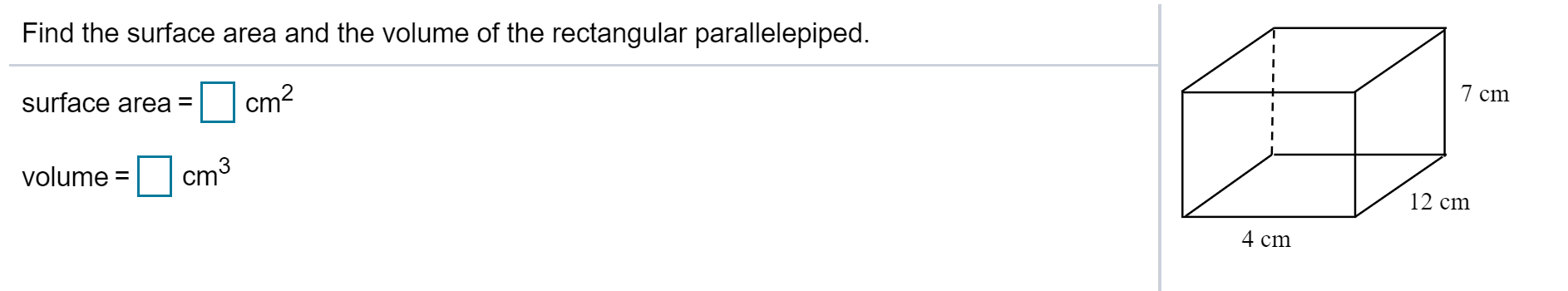# Find the surface area and the volume of the rectangular parallelepiped.cm27 cmsurface area =volume = cm3Ст312 cm4 cm

Question
30 viewshelp_outlineImage TranscriptioncloseFind the surface area and the volume of the rectangular parallelepiped. cm2 7 cm surface area = volume = cm3 Ст3 12 cm 4 cm fullscreen
check_circle

Step 1

Let l, w, and h be the length, width and height of the rectangular parallelepiped respectively.

From the figure,

The length, width and height of the rectangular parallelepiped is 4cm, 12cm, and 7cm respectively.

Step 2

The formula for the surface area of the rectangular parallelepiped is S=2lw+2wh+2lh and the volume of the rectangular parallelepiped is V=lwh.

Substitute l=4cm, w=12 cm, and h=7cm, we get

Step 3

and

...

### Want to see the full answer?

See Solution

#### Want to see this answer and more?

Solutions are written by subject experts who are available 24/7. Questions are typically answered within 1 hour.*

See Solution
*Response times may vary by subject and question.
Tagged in

### Geometry